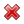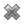# Fight Finance

#### CoursesTagsRandomAllRecentScoresScoreskeithphw $6,011.61 Jade$1,815.80 Chu $789.98 royal ne...$750.00 Leehy $713.33 Visitor$650.00 ZOE HY $640.00 JennyLI$625.61 Visitor $590.00 Visitor$555.33 Visitor $550.00 Visitor$550.00 Visitor $540.00 Visitor$500.00 Yizhou $489.18 Visitor$480.00 Visitor $480.00 Visitor$470.00 Visitor $464.70 Visitor$460.00

Question 121  capital structure, leverage, costs of financial distress, interest tax shield

Fill in the missing words in the following sentence:

All things remaining equal, as a firm's amount of debt funding falls, benefits of interest tax shields __________ and the costs of financial distress __________.

A four year bond has a face value of $100, a yield of 6% and a fixed coupon rate of 12%, paid semi-annually. What is its price? How is the AUD normally quoted in Australia? Using or terms? The market expects the Reserve Bank of Australia (RBA) to decrease the policy rate by 25 basis points at their next meeting. Then unexpectedly, the RBA announce that they will decrease the policy rate by 50 basis points due to fears of a recession and deflation. What do you expect to happen to Australia's exchange rate? The Australian dollar will: Calculate the price of a newly issued ten year bond with a face value of$100, a yield of 8% pa and a fixed coupon rate of 6% pa, paid semi-annually. So there are two coupons per year, paid in arrears every six months.

The Australian dollar's value was:

• 1.4875 USD per AUD on 31 August 1974.
• 0.4890 USD per AUD on 31 March 2001.

Did the Australian dollar or against the US dollar between these dates?

Which of the below formulas gives the payoff $(f)$ at maturity $(T)$ from being long a put option? Let the underlying asset price at maturity be $S_T$ and the exercise price be $X_T$.

Use the below information to value a levered company with constant annual perpetual cash flows from assets. The next cash flow will be generated in one year from now, so a perpetuity can be used to value this firm. Both the cash flow from assets including and excluding interest tax shields are constant (but not equal to each other).

 Data on a Levered Firm with Perpetual Cash Flows Item abbreviation Value Item full name $\text{CFFA}_\text{U}$ $100m Cash flow from assets excluding interest tax shields (unlevered) $\text{CFFA}_\text{L}$$112m Cash flow from assets including interest tax shields (levered) $g$ 0% pa Growth rate of cash flow from assets, levered and unlevered $\text{WACC}_\text{BeforeTax}$ 7% pa Weighted average cost of capital before tax $\text{WACC}_\text{AfterTax}$ 6.25% pa Weighted average cost of capital after tax $r_\text{D}$ 5% pa Cost of debt $r_\text{EL}$ 9% pa Cost of levered equity $D/V_L$ 50% pa Debt to assets ratio, where the asset value includes tax shields $t_c$ 30% Corporate tax rate

What is the value of the levered firm including interest tax shields?

Below is a graph of 3 peoples’ utility functions, Mr Blue (U=W^(1/2) ), Miss Red (U=W/10) and Mrs Green (U=W^2/1000). Assume that each of them currently have \$50 of wealth.Which of the following statements about them is NOT correct?

(a) Mr Blue would prefer to invest his wealth in a well diversified portfolio of stocks rather than a single stock, assuming that all stocks had the same total risk and return.

Question 844  gross domestic product deflator, consumer price index, inflation, no explanation

An Australian-owned company produces milk in New Zealand and exports all of it to China. If the price of the milk increases, which of the following would increase?